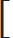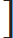Arithmetic Progression: x, x + y, x + 2y, x + 3y are said to be in A.P. Here x is first term and common difference is y

in Other
Q:

Arithmetic Progression: x, x + y, x + 2y, x + 3y are said to be in A.P. Here x is first term and common difference is y

a) nth term = x + (n – 1) y

 b) Sum of n terms = n [2x + (n – 1)y] 2

 3) 1 + 2 + 3 ± - - - - + n = n(n + 1) 2

 4) (12 + 22 + 32 - - - - + n2) = n(n + 1) (2n + 1) 6

 5) (13 + 23 + 33 - - - - + n3) =n(n + 1)2 2